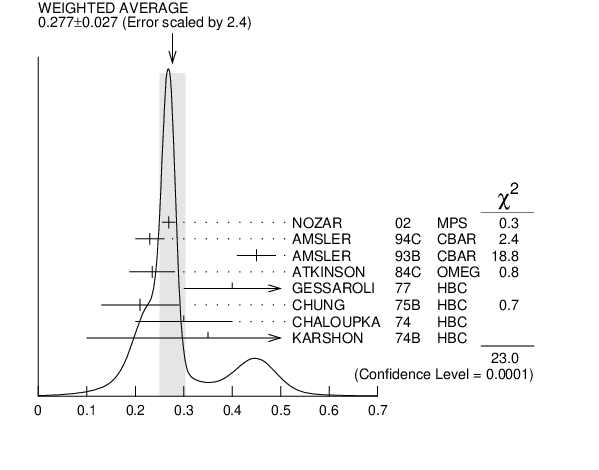#### ${{\boldsymbol b}_{{1}}{(1235)}}{\boldsymbol D}{\mathrm -wave}/{\boldsymbol S}{\mathrm -wave}$ AMPLITUDE RATIO IN DECAY OF ${{\boldsymbol b}_{{1}}{(1235)}}$ $\rightarrow$ ${{\boldsymbol \omega}}{{\boldsymbol \pi}}$

VALUE EVTS DOCUMENT ID TECN CHG  COMMENT
 $\bf{ 0.277 \pm0.027}$ OUR AVERAGE  Error includes scale factor of 2.4. See the ideogram below.
$0.269$ $\pm0.009$ $\pm0.010$
 2002
MPS - 18 ${{\mathit \pi}^{-}}$ ${{\mathit p}}$ $\rightarrow$ ${{\mathit \omega}}{{\mathit \pi}^{-}}{{\mathit p}}$
$0.23$ $\pm0.03$
 1994 C
CBAR $0.0$ ${{\overline{\mathit p}}}$ ${{\mathit p}}$ $\rightarrow$ ${{\mathit \omega}}{{\mathit \eta}}{{\mathit \pi}^{0}}$
$0.45$ $\pm0.04$
 1993 B
CBAR $0.0$ ${{\overline{\mathit p}}}$ ${{\mathit p}}$ $\rightarrow$ ${{\mathit \omega}}{{\mathit \pi}^{0}}{{\mathit \pi}^{0}}$
$0.235$ $\pm0.047$
 1984 C
OMEG 20$-$70 ${{\mathit \gamma}}{{\mathit p}}$
$0.4$ ${}^{+0.1}_{-0.1}$
 1977
HBC - 11 ${{\mathit \pi}^{-}}$ ${{\mathit p}}$ $\rightarrow$ ${{\mathit \pi}^{-}}{{\mathit \omega}}{{\mathit p}}$
$0.21$ $\pm0.08$
 1975 B
HBC + 7.1 ${{\mathit \pi}^{+}}{{\mathit p}}$
$0.3$ $\pm0.1$
 1974
HBC - 3.9$-$7.5 ${{\mathit \pi}^{-}}{{\mathit p}}$
$0.35$ $\pm0.25$ 600
 1974 B
HBC + 4.9 ${{\mathit \pi}^{+}}{{\mathit p}}$${{\mathit b}_{{1}}{(1235)}}{\mathit D}{\mathrm -wave}/{\mathit S}{\mathrm -wave}$ amplitude ratio in decay of ${{\mathit b}_{{1}}{(1235)}}$ $\rightarrow$ ${{\mathit \omega}}{{\mathit \pi}}$
References:
 NOZAR 2002
PL B541 35 A Study of the Reaction ${{\mathit \pi}^{-}}$ ${{\mathit p}}$ $\rightarrow$ ${{\mathit \omega}}{{\mathit \pi}^{-}}{{\mathit p}}$ at 18-GeV/$\mathit c$: The D and S Decay Amplitudes for ${{\mathit b}_{{1}}{(1235)}}$ $\rightarrow$ ${{\mathit \omega}}{{\mathit \pi}}$
 AMSLER 1994C
PL B327 425 Study of ${{\overline{\mathit p}}}{{\mathit p}}$ Annihilation at Rest into ${{\mathit \omega}}{{\mathit \eta}}{{\mathit \pi}^{0}}$
 AMSLER 1993B
PL B311 362 Antiproton Proton Annihilation at Rest into ${{\mathit \omega}}{{\mathit \pi}^{0}}{{\mathit \pi}^{0}}$
 ATKINSON 1984C
NP B243 1 A Spin Parity Analysis of the ${{\mathit \omega}}{{\mathit \pi}^{0}}$ Enhancement Photoproduced in the Energy Range 20 to 70 GeV
 GESSAROLI 1977
NP B126 382 Analysis of the ${{\mathit \omega}}{{\mathit \pi}^{-}}$ System Produced in the Reaction ${{\mathit \pi}^{-}}$ ${{\mathit p}}$ $\rightarrow$ ${{\mathit \pi}^{-}}{{\mathit \pi}^{-}}{{\mathit \pi}^{+}}{{\mathit \pi}^{0}}$ ${{\mathit p}}$ at 11.2 ${\mathrm {GeV/}}\mathit c$
 CHUNG 1975B
PR D11 2426 Spin Parity Analysis of the ${{\mathit B}}$ Meson
 CHALOUPKA 1974
PL 51B 407 Production and Decay Properties of the ${{\mathit B}}$ Meson
 KARSHON 1974B
PR D10 3608 Production and Decay Mechanism of the ${{\mathit B}}$ Meson and the ${{\mathit \omega}}{{\mathit \pi}}$ System in ${{\mathit \pi}^{+}}{{\mathit p}}$ Interactions at 5 ${\mathrm {GeV/}}\mathit c$